# Polyhedron

Go back to  'Geometry'

 1 Introduction to Polyhedrons 2 Types of Polyhedrons 3 Tips and Tricks 4 What are the 5 Polyhedrons? 5 Common Polyhedra 6 Counting Faces, Vertices, and Edges of Polyhedron 7 Important Notes on Polyhedrons 8 Solved Examples of Polyhedrons 9 Practice Questions on Polyhedrons 10 Maths Olympiad Sample Papers 11 Frequently Asked Questions (FAQs)

We at Cuemath believe that Math is a life skill. Our Math Experts focus on the “Why” behind the “What.” Students can explore from a huge range of interactive worksheets, visuals, simulations, practice tests, and more to understand a concept in depth.

Book a FREE trial class today! and experience Cuemath’s LIVE Online Class with your child.

## Introduction to Polyhedrons

### What are polyhedrons?

A polyhedron is a three-dimensional shape that has flat faces, straight edges, and sharp corners or vertices.

The word "polyhedron" is derived from the Greek words poly which means "many" and hedron which means "surface".

Thus, polyhedron means many flat surfaces joined together to form a 3-dimensional shape.

The plural of a polyhedron is polyhedra.

Depending on the number of polygon faces in a polyhedron, they are classified into various polyhedron shapes.

The various polyhedron shapes are also defined by their polygon bases.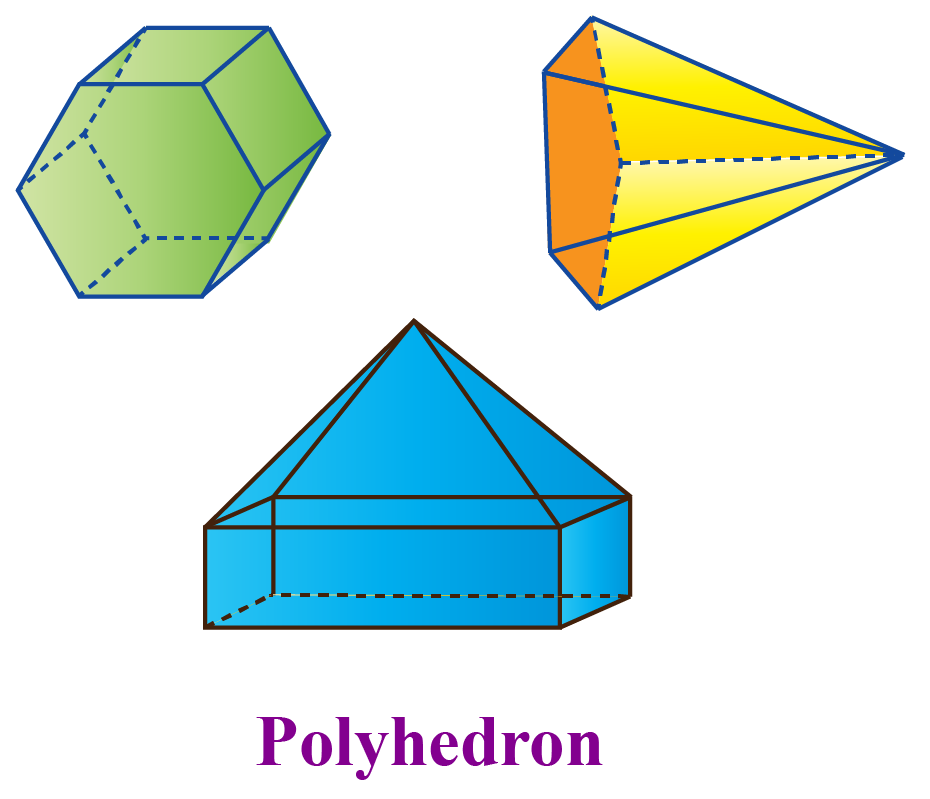Our lives revolve around polyhedra as we live in a three-dimensional world.

For instance, bricks, dice, office tables, houses, and so on are all examples of polyhedra.

### Polyhedron Shapes

Based on the number of polygonal faces and bases, polyhedrons are classified into various polyhedron shapes.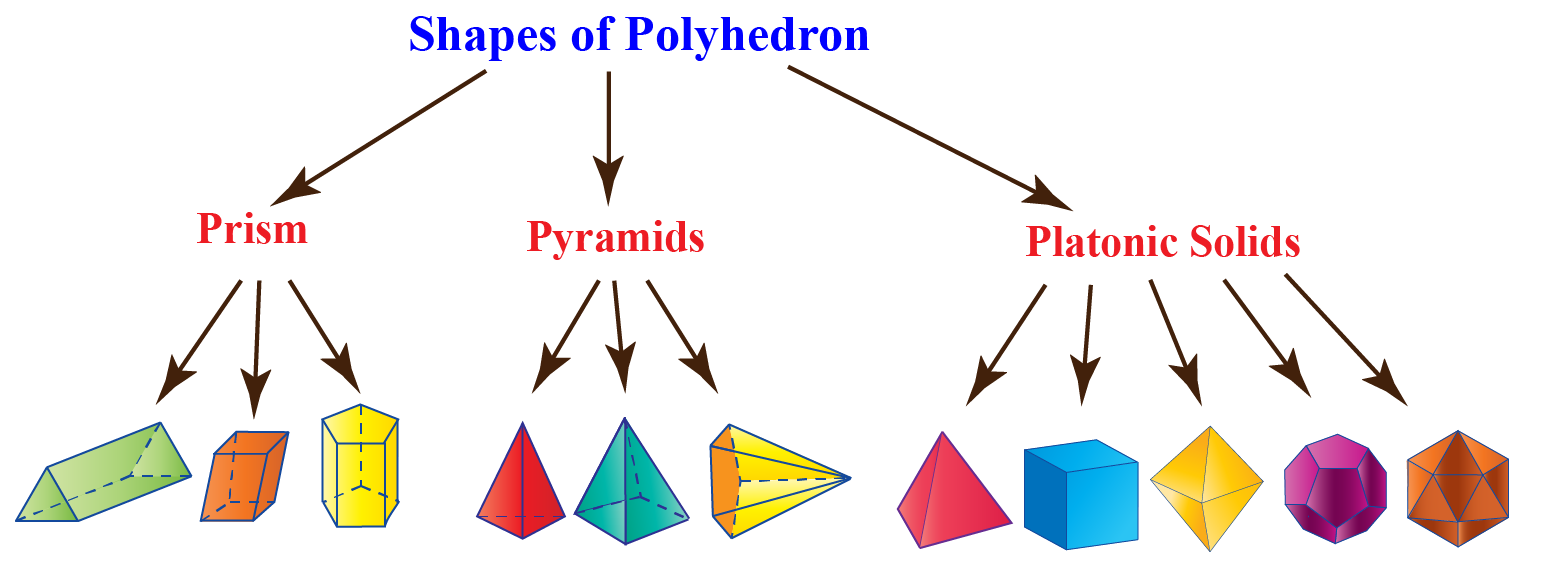## Types of Polyhedrons

There are three main categories under polyhedra.

• Prism family
• Pyramid family
• Platonic solids

These are again mainly divided into two types - Regular polyhedra and Irregular polyhedra.

### Regular Polyhedra

A polyhedron whose faces are all regular polygons and are congruent to each other, and have the same number of faces meeting at each vertex is known as a regular polyhedron.

In a regular polyhedron, the polyhedral angles are all equal.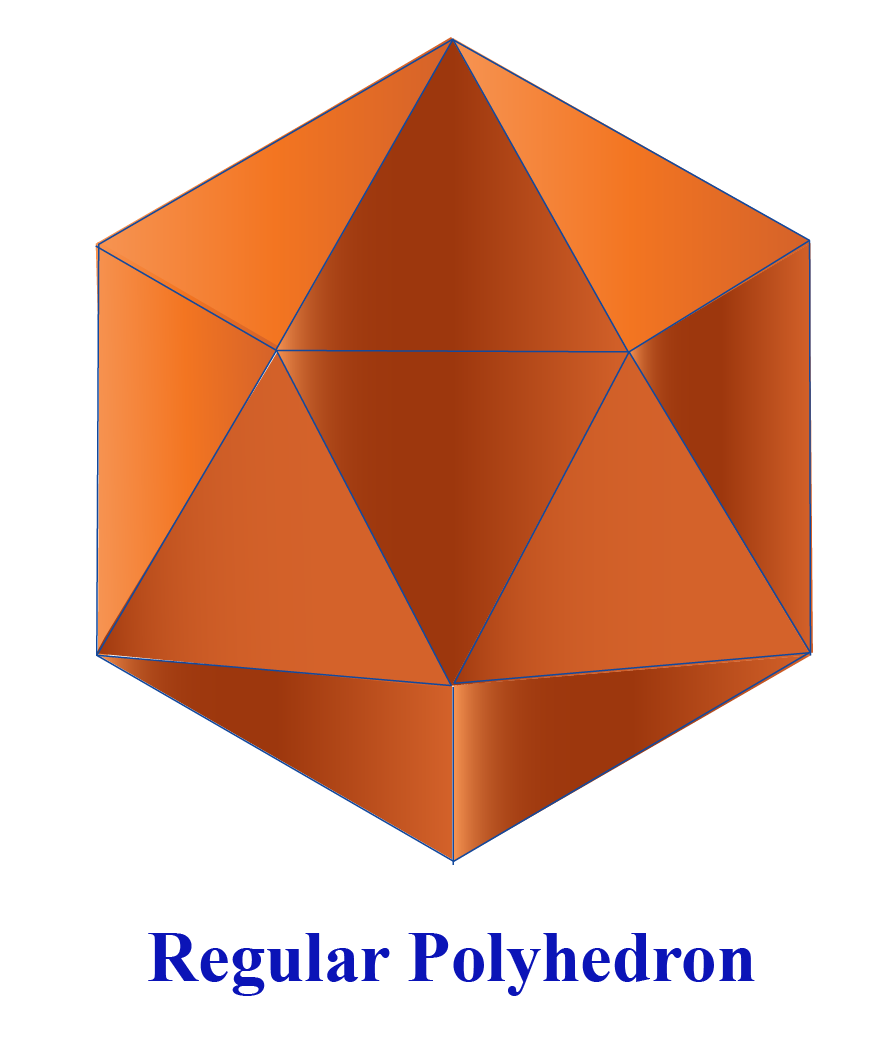### Irregular Polyhedra

A polyhedron with irregular polygonal faces that are not congruent to each other, and in which the polyhedral angles are not equal is called an irregular polyhedron.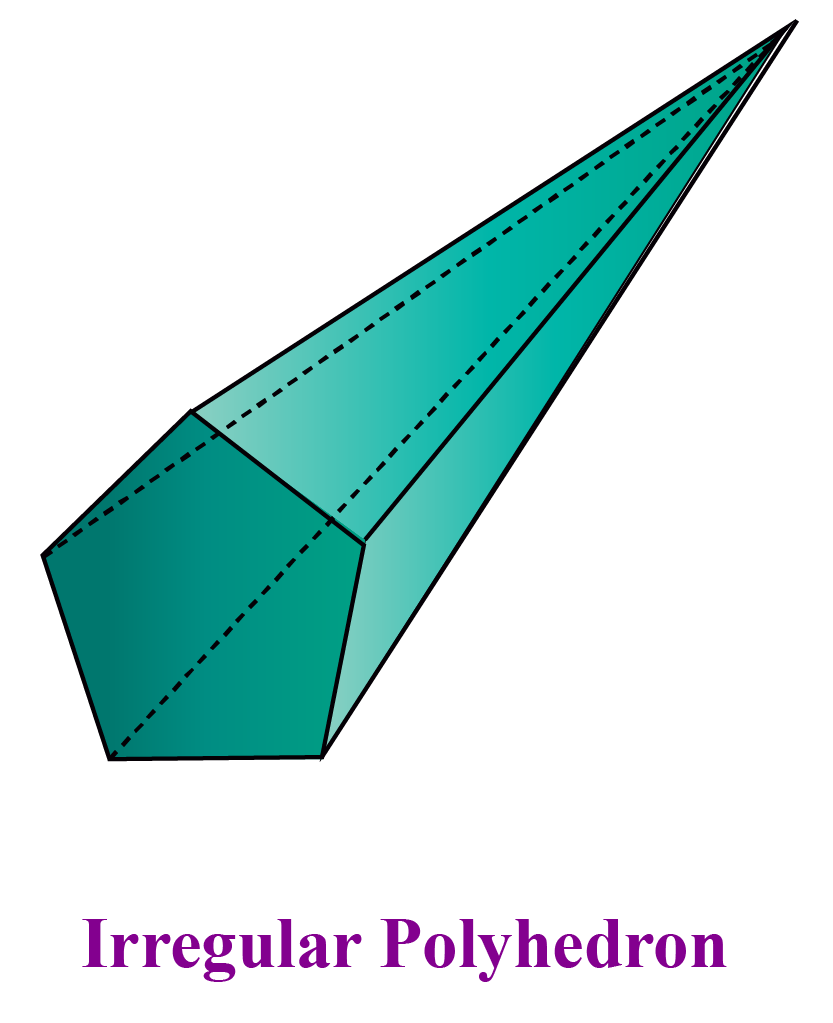### Concave Polyhedron

If a line segment joining two points lies on the bulged surface of a polyhedron, it is called a Concave polyhedron.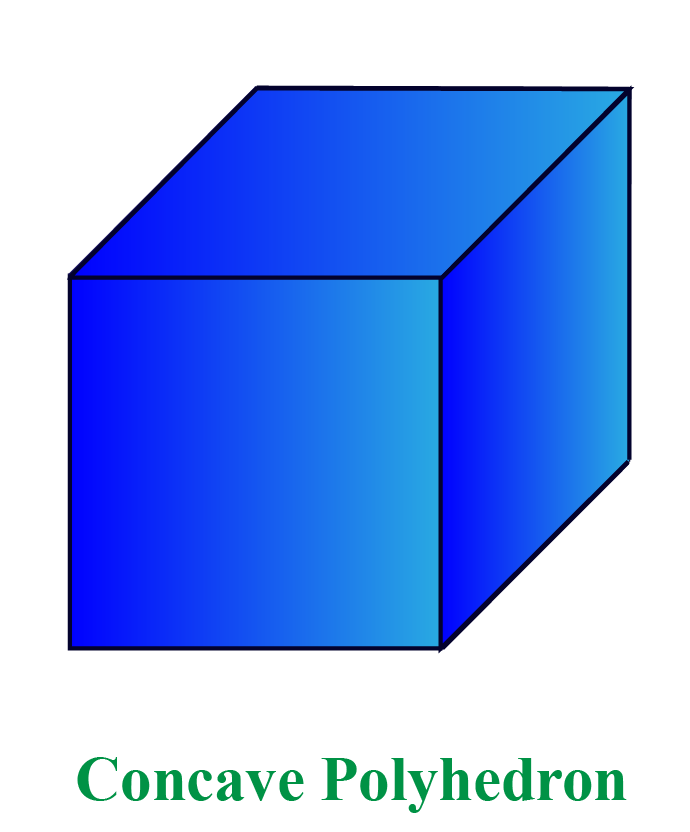### Convex Polyhedron

If a line segment joining two points lies on the inside surface of the polyhedron, it is called a Convex polyhedron.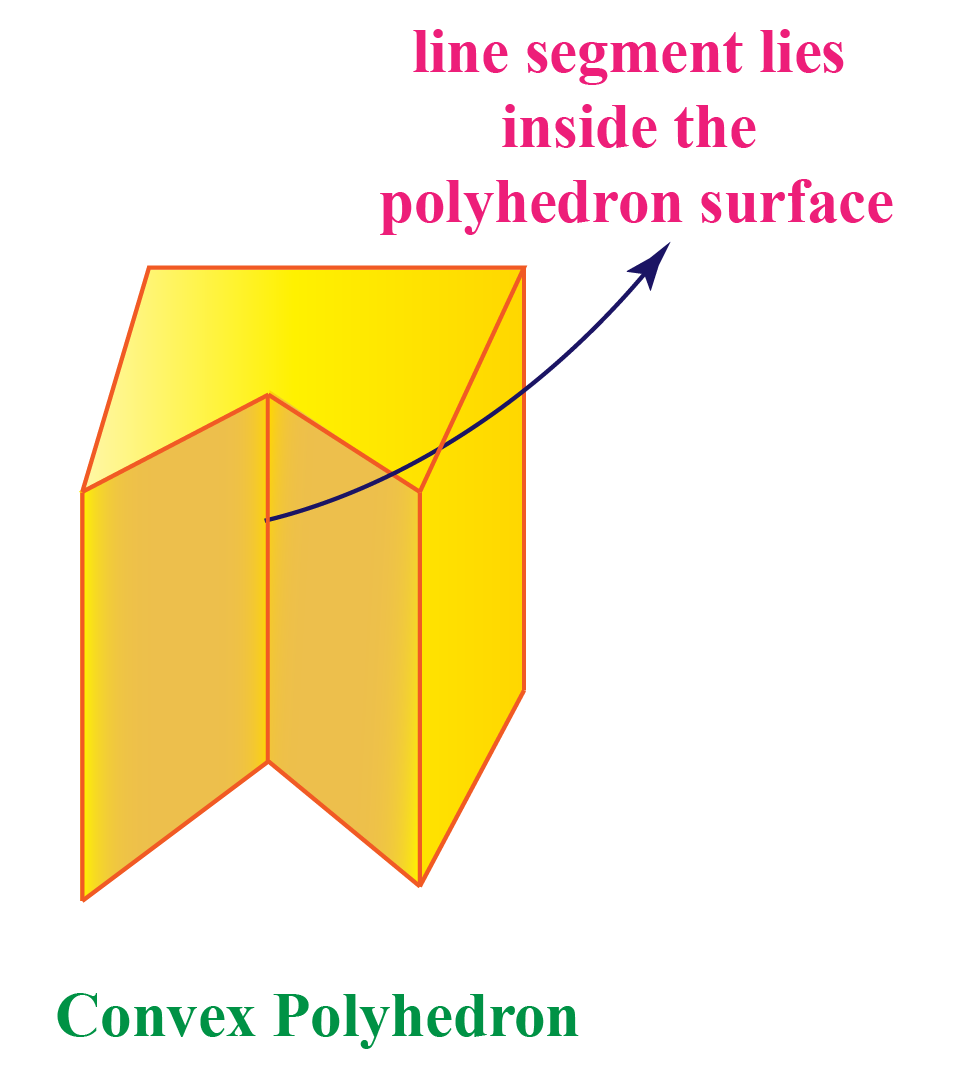## What are the 5 Polyhedrons?

The regular polyhedra or platonic solids are convex with the same number of faces meeting at each vertex, and sides that are congruent.

They are 5 regular polyhedra.

1. Tetrahedron
2. Cube
3. Octahedron
4. Dodecahedron
5. Icosahedron

### Tetrahedron

• A tetrahedron is a solid shape that has 4 faces.
• with each side having an equilateral triangles.
• which consists of 4 edges and 4 vertices.
• Surface area of tetrahedron   =$$\sqrt{3}\:a^2$$
• The volume of the tetrahedron = \begin{align}\frac{\sqrt{2}}{12}\:a^3\end{align}

### Cube

• A cube has 6 faces.
• Each face is in the shape of a square.
• It has 12 edges.
• It has 8 vertices and 3 edges meet at each vertex.
• Surface area of a cube = $$6\:a^2$$
• Volume of a cube = $$a^3$$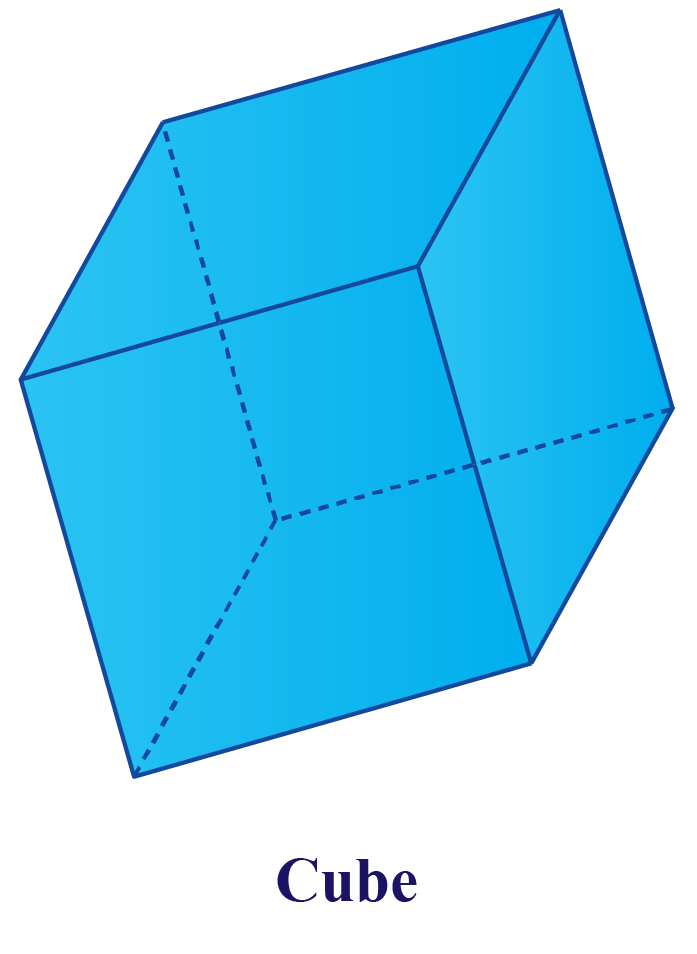### Octahedron

• An octahedron has 8 faces.
• Each face is an equilateral triangle.
• It has 12 edges.
• It has 6 vertices and 4 edges meet at each vertex.
• Surface area of an octahedron = $$2 \sqrt{3}\:a^2$$
• Volume of an octahedron = \begin{align}\frac{\sqrt{2}}{3}\:a^3\end{align}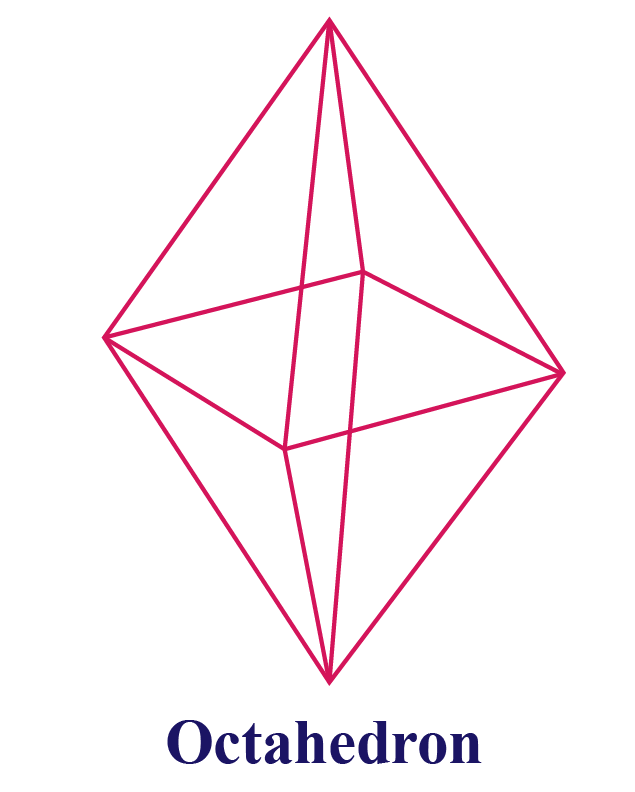### Dodecahedron

• The dodecahedron consists of 12 faces.
• Each face is in the shape of a regular pentagon.
• It has 30 edges.
• It has 20 vertices and 3 edges meet at each vertex.
• Surface area of a dodecahedron = $$3\sqrt{25+10\sqrt{5}}\:a^2$$
• Volume of a dodecahedron = \begin{align}\frac{15+7\sqrt{5}}{4}\:a^3\end{align}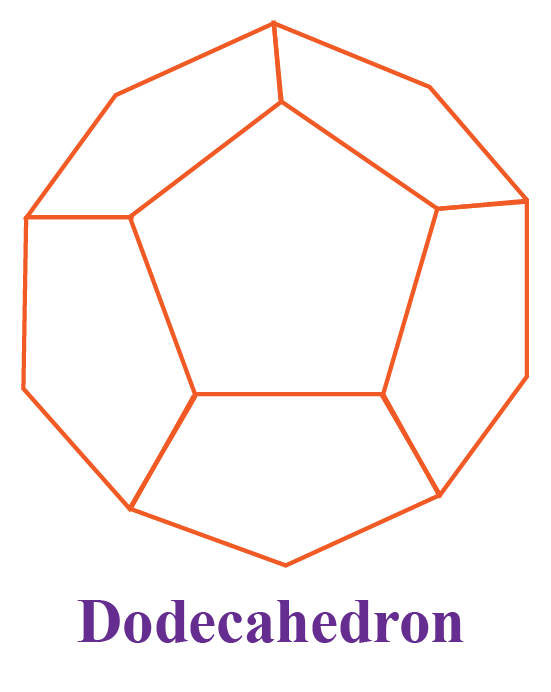### Icosahedron

• This polyhedron has 20 faces.
• Each face is in the shape of an equilateral triangle.
• It has 30 edges.
• It has 12 vertices and 5 edges meet at every vertex.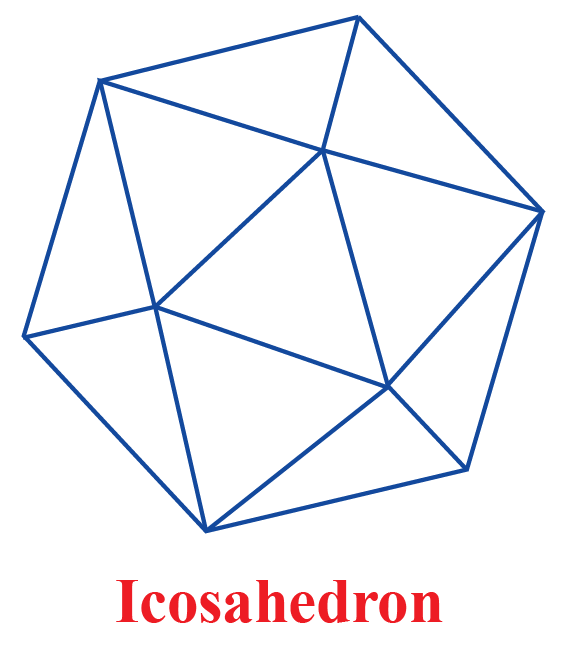## Common Polyhedra

The plural of a polyhedron is polyhedrons or polyhedra.

The most commonly found polyhedra are:

• Platonic solids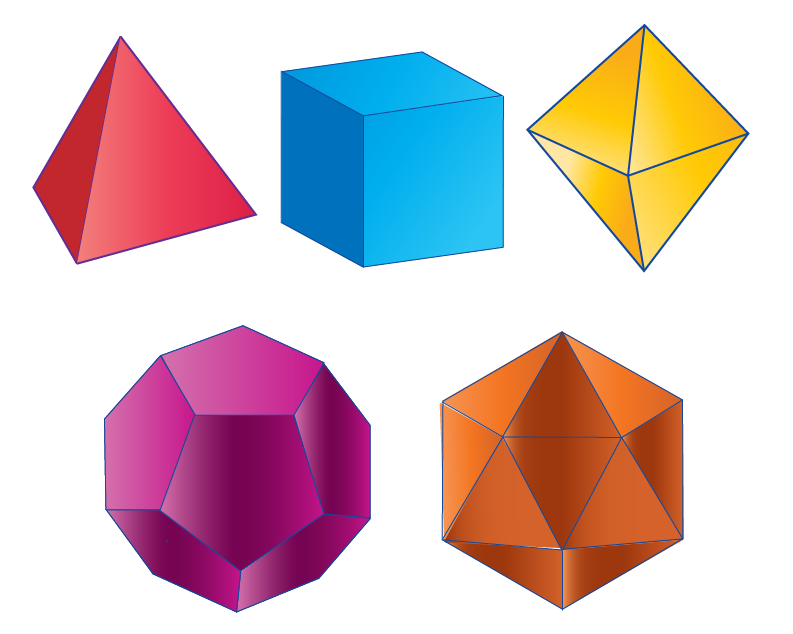• Prisms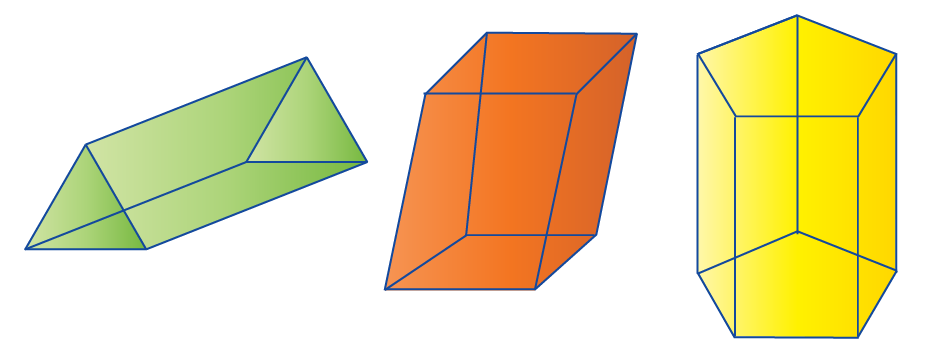• Pyramids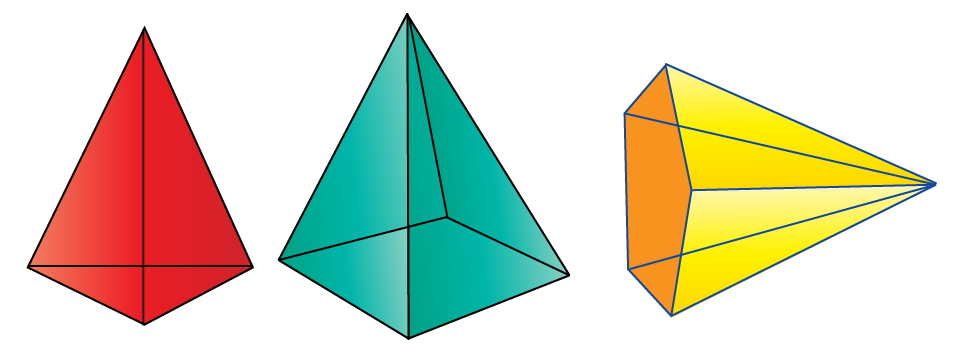###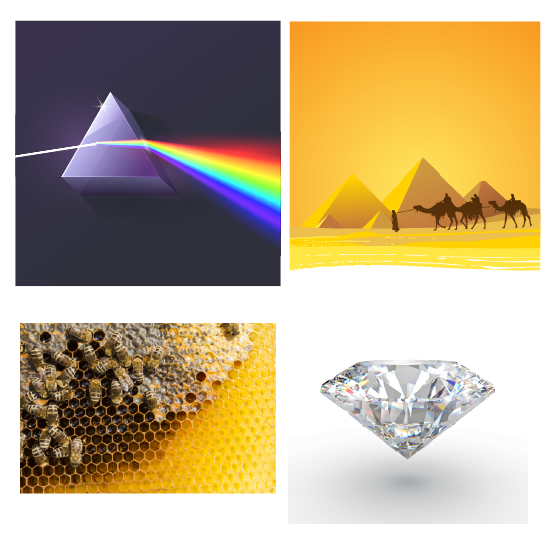Prisms, Egyptian pyramids, beehives, diamonds are some real-life examples of polyhedra.

CLUEless in Math? Check out how CUEMATH Teachers will explain Polyhedron to your kid using interactive simulations & worksheets so they never have to memorise anything in Math again!

Explore Cuemath Live, Interactive & Personalised Online Classes to make your kid a Math Expert. Book a FREE trial class today!

## Counting Faces, Vertices, and Edges

The faces of polyhedrons are the flat sides of the individual polyhedral geometry.

The edges indicate where two or more faces meet.

The vertices indicate the corners.

### Counting Faces

Look at the shape below.

How many faces can you count?

Can you see that it has a face on the top, on the bottom, to the left, to the right, on the front and at the back?

In this example, we can observe 8 faces.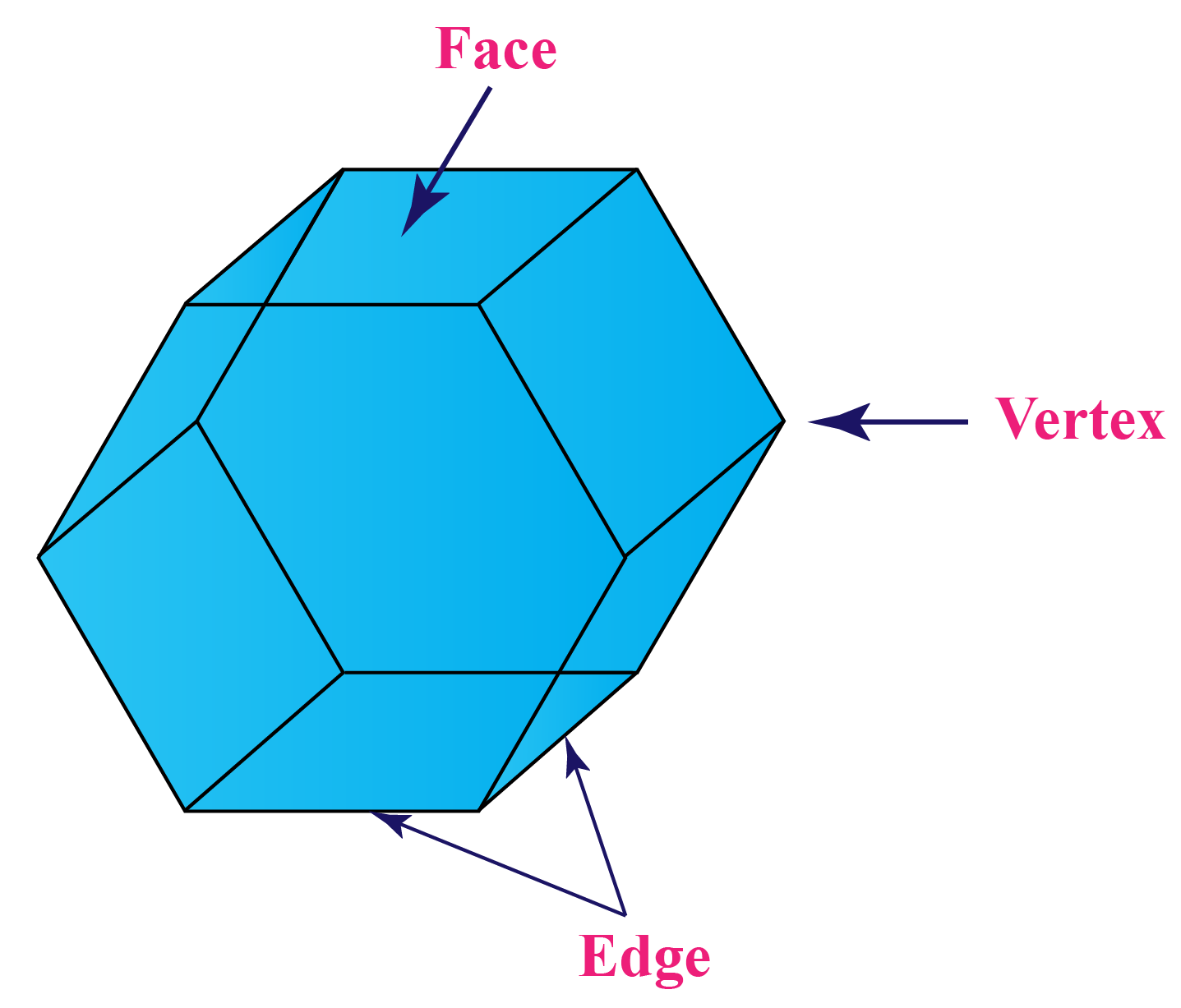### Counting Edges

Now, let us count the edges in the above polyhedron.

We can observe that the shape has 18 edges.

### Counting Vertices

You can observe that there are 12 vertices in this shape.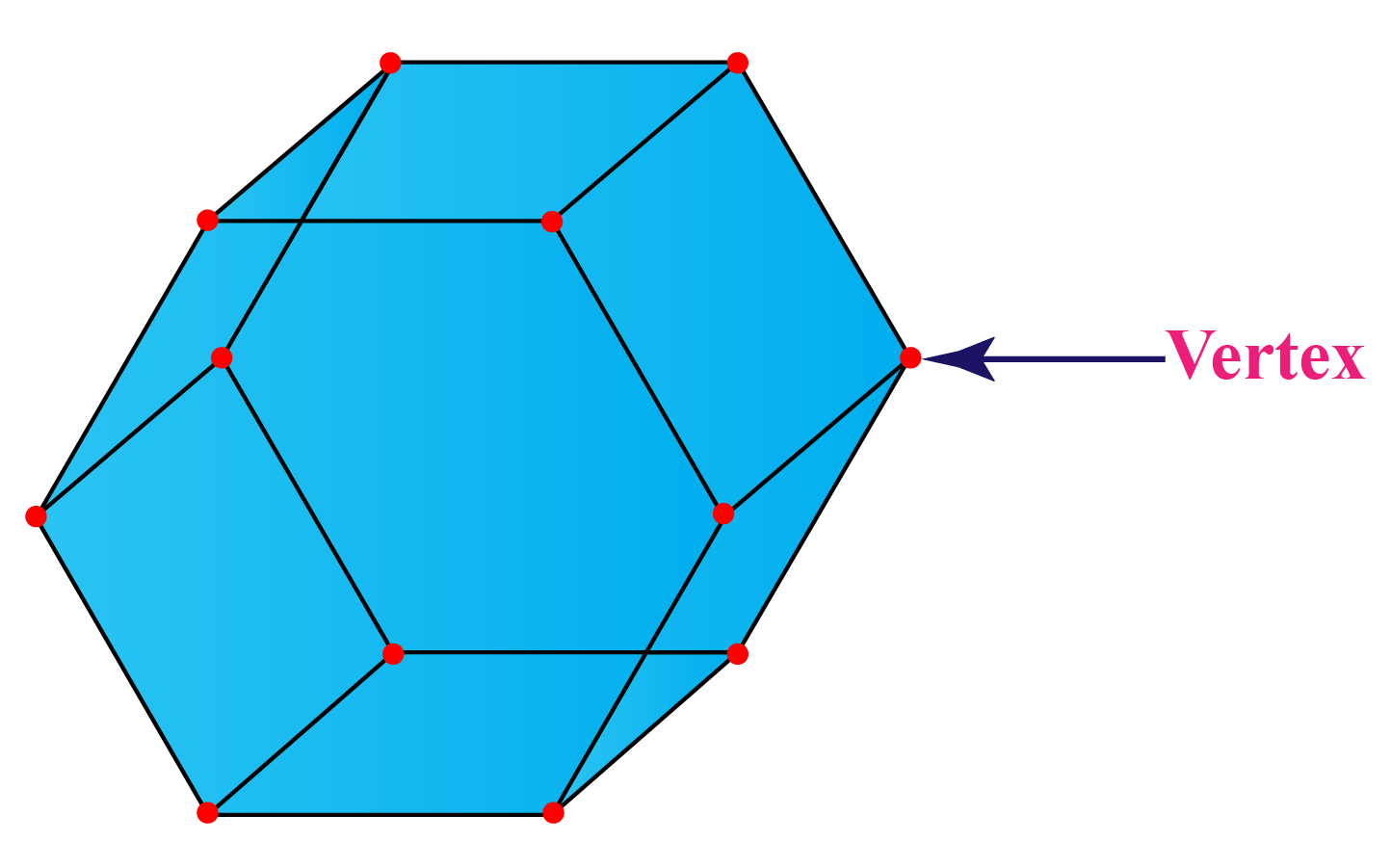### The Relationship

There is a relationship between the number of faces, edges, and vertices in a polyhedron.

We can represent this relationship as a math formula.

This relationship is known as Euler's Formula.

 F + V - E = 2

where,

F = Number of faces

V = Number of vertices

E = Number of edges

### Polyhedron Formula

In the simulation shown below, you can explore the relationship between the faces, vertices, and edges of a tetrahedron using Euler's formula.Important Notes
1. A polyhedron is a three-dimensional shape with flat faces, straight edges and sharp vertices.
2. They are usually found as prisms, pyramids, and platonic solids.
3. The 5 regular polyhedra or platonic solids are - Tetrahedron, Cube, Octahedron, Dodecahedron, and Icosahedron.
4. By counting the number of faces, vertices, and edges of a polyhedron shape, we can relate them with Euler's formula, F + V - E = 2

Help your child score higher with Cuemath’s proprietary FREE Diagnostic Test. Get access to detailed reports, customised learning plans and a FREE counselling session. Attempt the test now.

## Solved Examples of Polyhedrons

 Example 1

In which of the following regular polyhedrons do 3 pentagons meet at each vertex?

(a)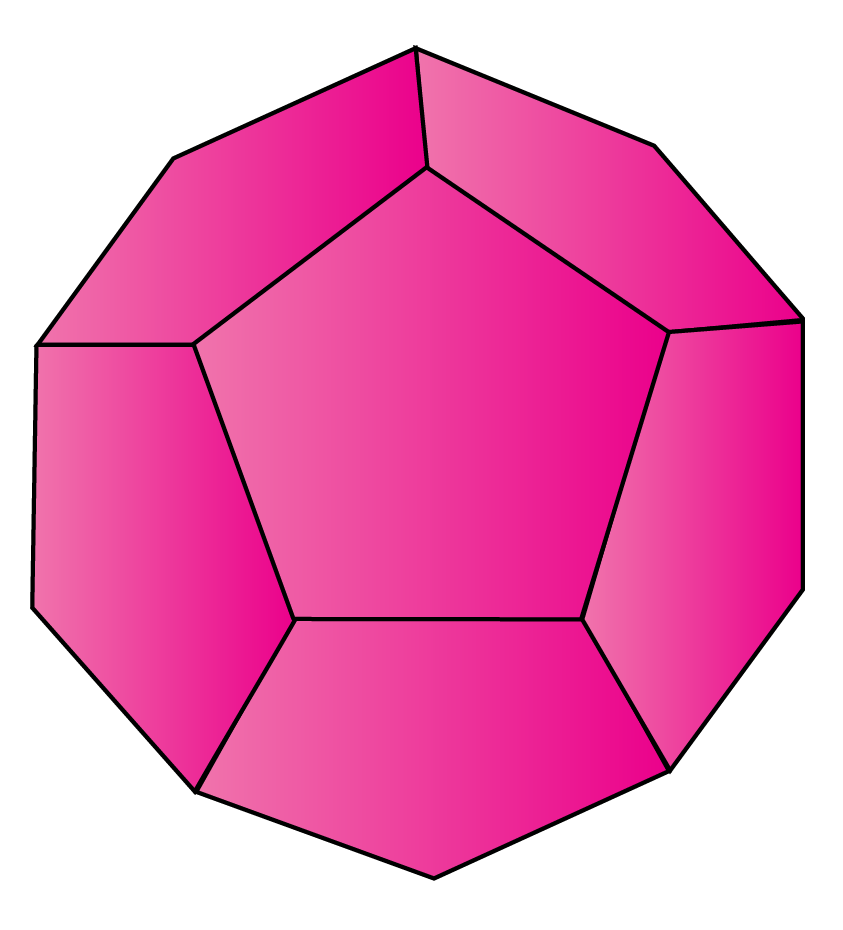(b)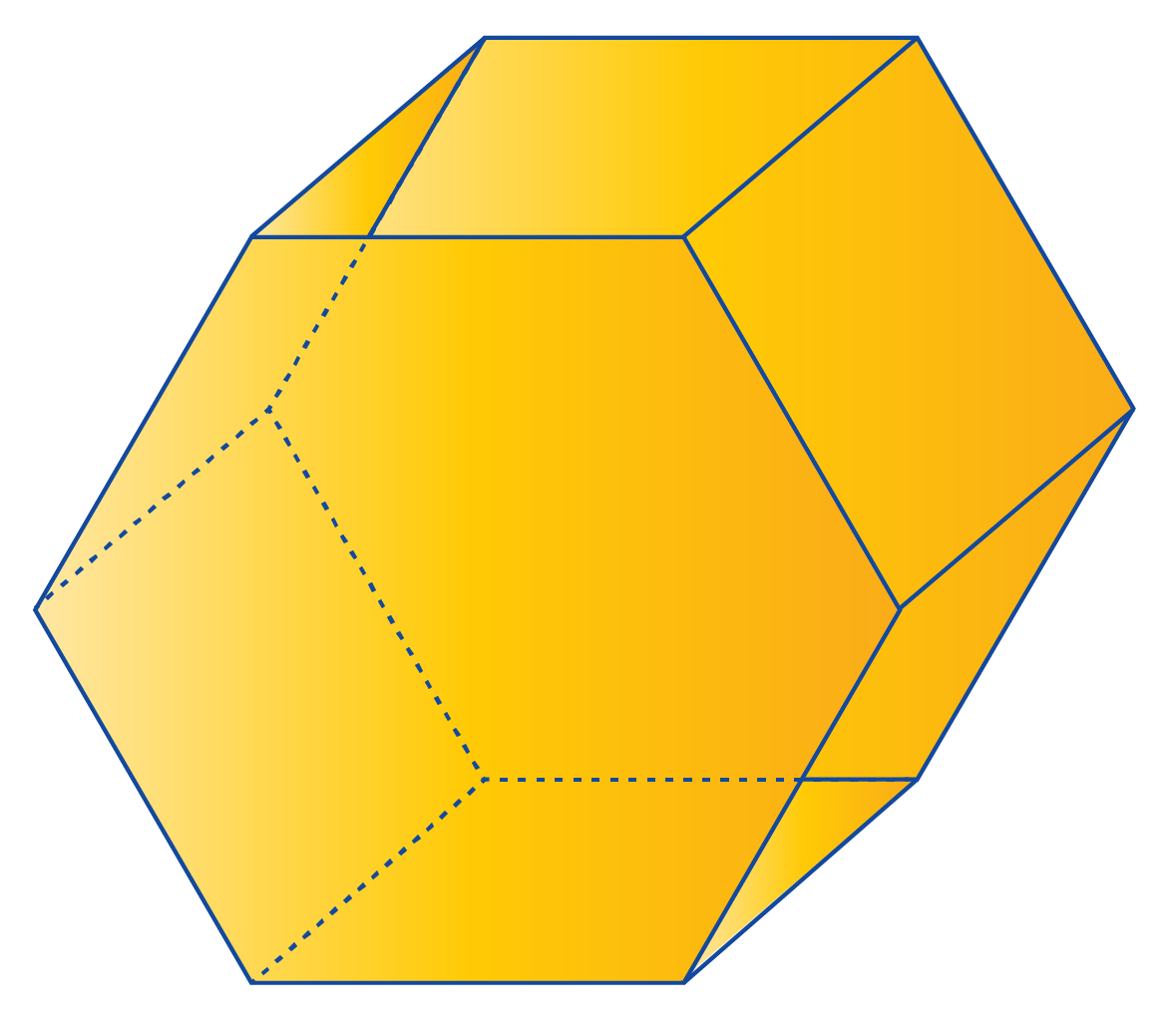(c)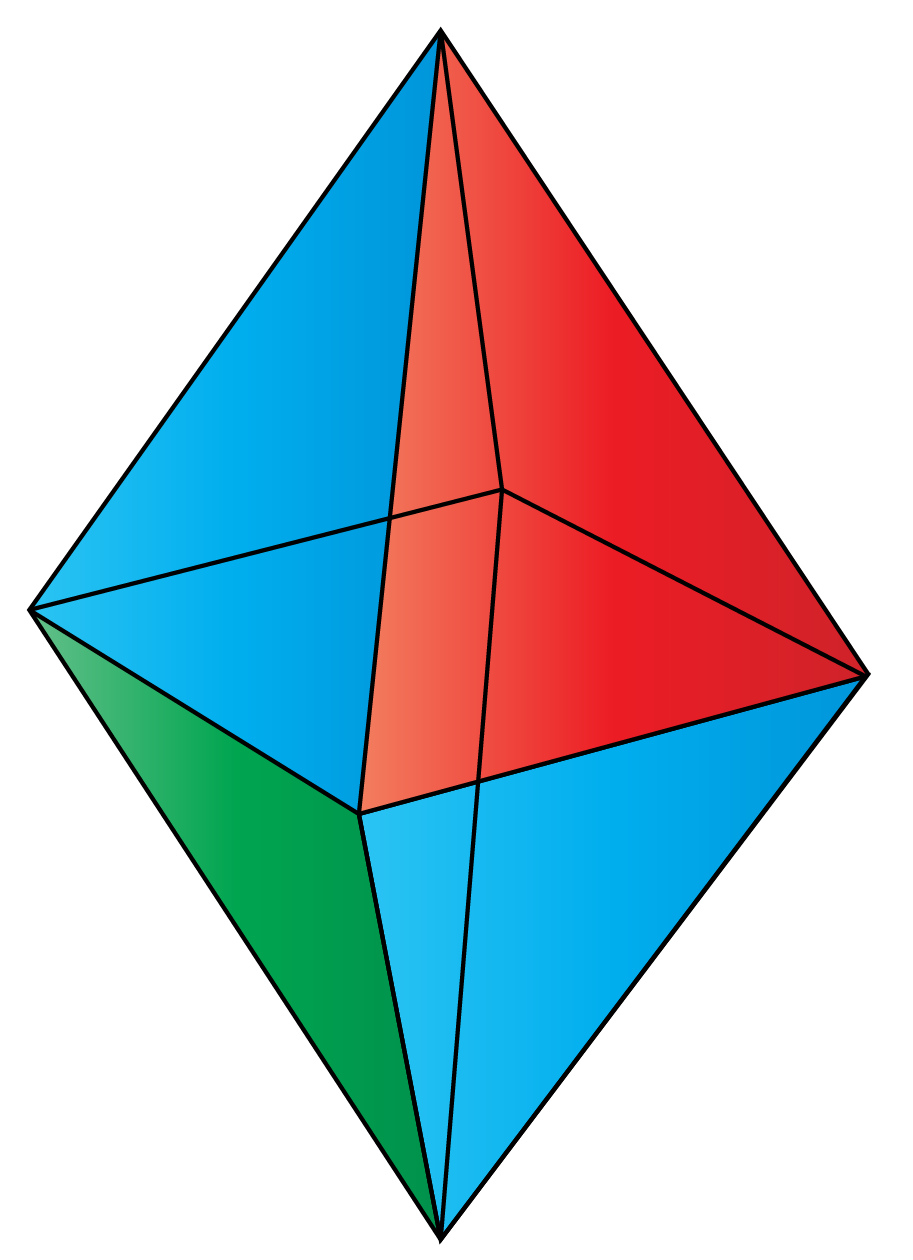Solution:

(a) This polyhedron has 3 pentagons meeting at each vertex.

(b) This polyhedron has 2 rectangles and one heptagon meeting at each vertex.

(c) This polyhedron has 3 triangles meeting at the vertex.

 $$\therefore$$ 3 pentagons meet at each vertex in (a)
 Example 2

Mary counted the edges, faces, and vertices of a polyhedron.

She states that there are 12 vertices, 5 faces, and 10 edges.

Did she make an error?

Solution:

Using Euler's formula, we can verify whether she counted them correctly or not.

According to her, the number of faces are 5

The number of vertices are 12

The number of edges are 10

Substituting these numbers into Euler's Formula:

 F + V - E = 2

$F+V-E = 2$

$5+12-10 = 2$

$7 \neq 2$

 $$\therefore$$ Mary has made an error while counting.
 Example 3

Which of the following are platonic solids?

(a) Cylinder

(b) Cone

(c) Octahedron

(d) Triangular Prism

Solution:

The commonly found platonic solids or regular polyhedrons are:

1. Tetrahedron
2. Cube
3. Octahedron
4. Dodecahedron
5. Icosahedron
 $$\therefore$$ (c) Octahedron
 Example 4

What is the surface area for the below polyhedron?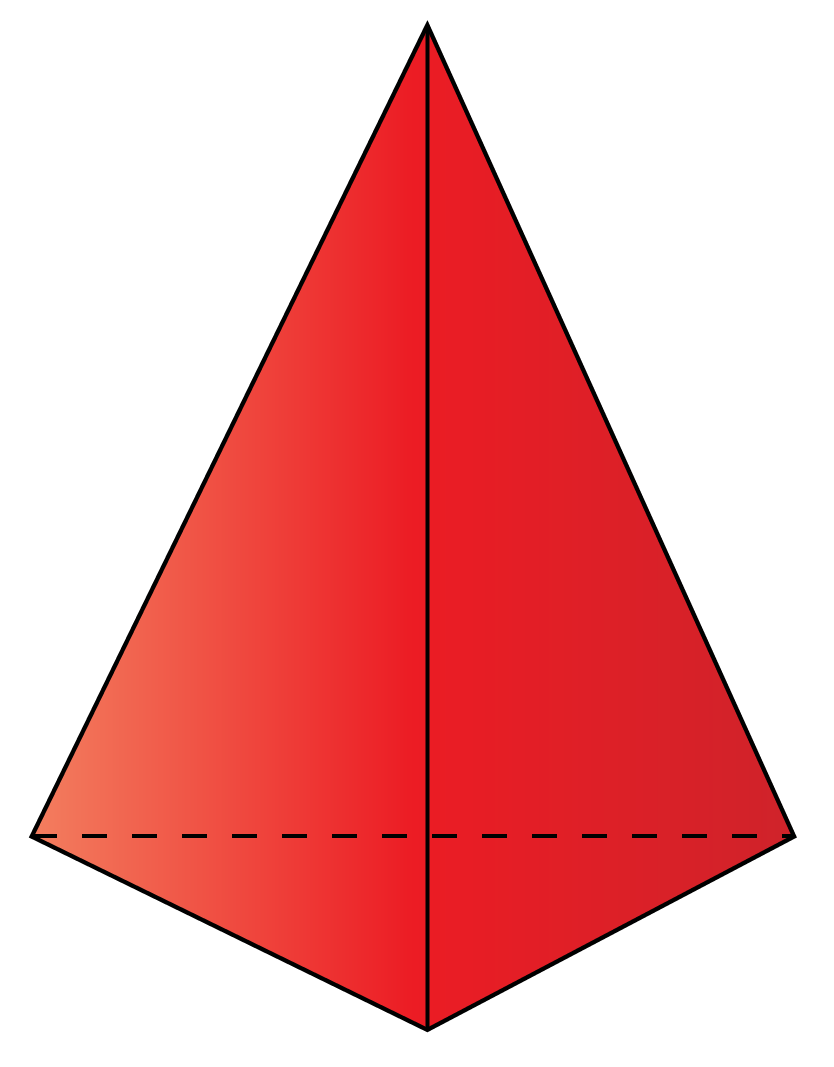(a) $$6\:e^2$$

(b) $$2 \sqrt{3}\:e^2$$

(c) $$\sqrt{3}\:e^2$$

(d) $$3\sqrt{25+10\sqrt{5}}\:e^2$$

Solution:

(c)  The surface area of Tetrahedron is $$\sqrt{3}\:e^2$$

 $$\therefore$$ SA of Tetrahedron  = $$\sqrt{3}\:e^2$$
 Example 5

On the way home from school, Henry found a solid with six faces, six vertices, and ten edges.

Identify this polyhedron.

Solution: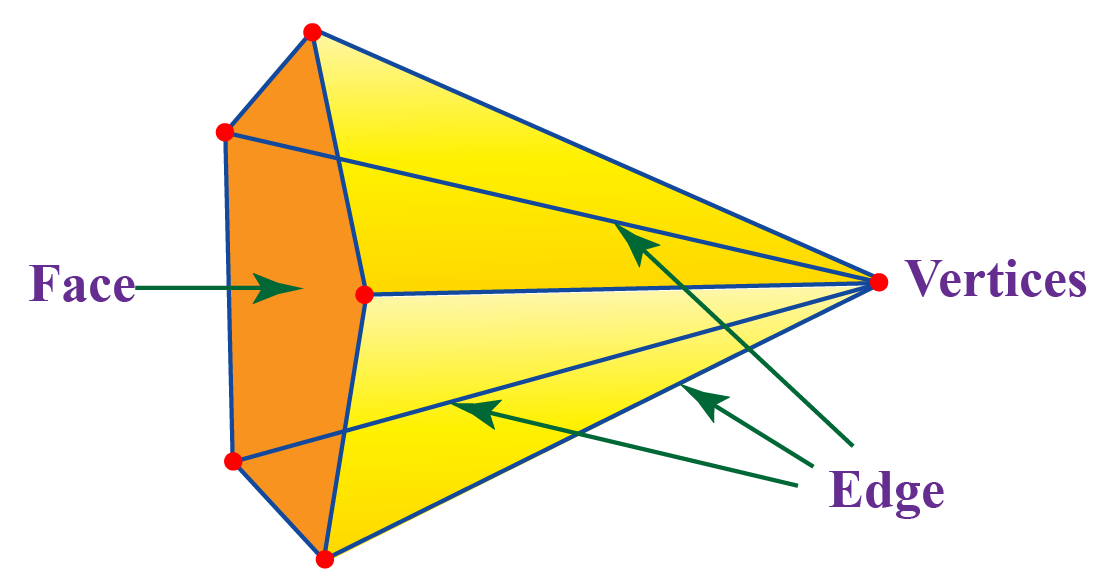From the above figure, it is clear that only a pentagonal pyramid satisfies the given parameters.

 $$\therefore$$ Henry found a pentagonal pyramid.

## Practice Questions on Polyhedrons

Here are a few activities for you to practice.

IMO (International Maths Olympiad) is a competitive exam in Mathematics conducted annually for school students. It encourages children to develop their math solving skills from a competition perspective.

## 1. How many faces does a polyhedron have?

Depending on the number of flat sides of the polygonal based polyhedron,we can count the number of faces.

We can also find the number of faces using Euler's formula, F + V - E = 2, if we know the number of edges and vertices.

## 2. Is sphere a polyhedron?

No, a sphere is a curved surface whereas polyhedrons only have straight and flat surfaces.

## 3. Is prism a polyhedron?

Yes, it is a polyhedron.

A prism has flat faces, straight edges, and sharp vertices.

Triangular prism, rectangular prism, and pentagonal prism come under polyhedrons.

## 4. What is not a polyhedron?

Three-dimensional shapes that have curved faces and which are not polygons are not classified as polyhedrons.

Example: Cone, cylinder, torus are not polyhedrons.

More Important Topics
Numbers
Algebra
Geometry
Measurement
Money
Data
Trigonometry
Calculus